Pre-Algebra 1
Set 1 (10 Questions)
Algebra and Functions
Write and evaluate an algebraic expression for a given situation, using up to three variables.
Apply algebraic order of operations and the commutative, associative, and distributive properties to evaluate expressions; and justify each step in the process.
Solve problems manually by using the correct order of operations or by using a scientific calculator.
From Mr. Anker Tests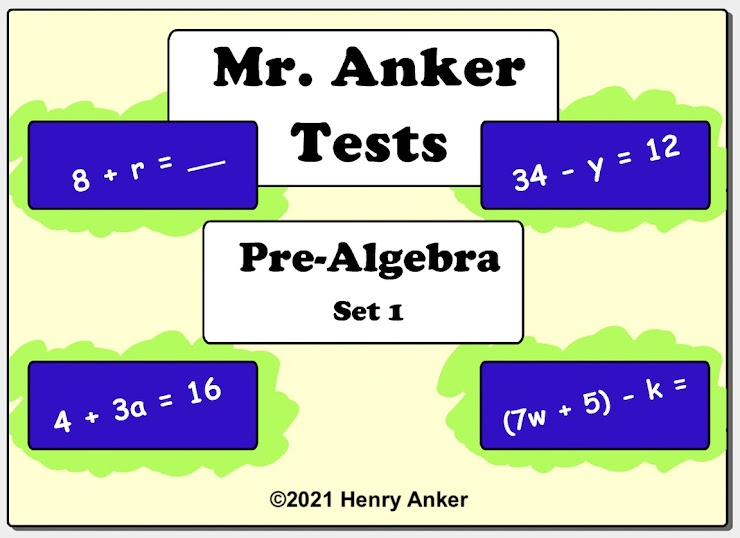1. Answer the question below. *
1 point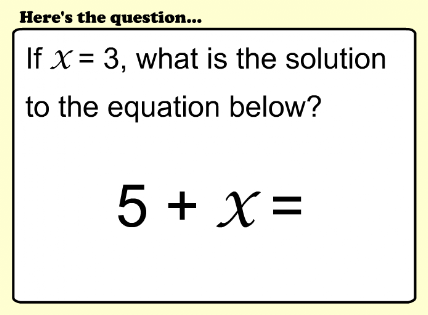2. Answer the question on the line below. *
1 point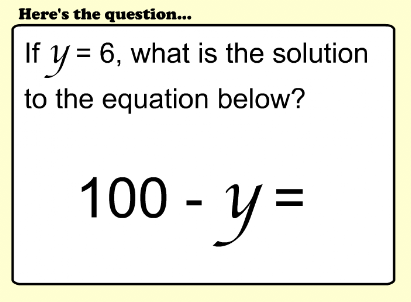3. Answer the question below. *
1 point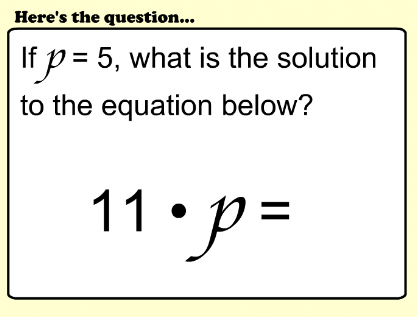4. Answer the question on the line below. *
1 point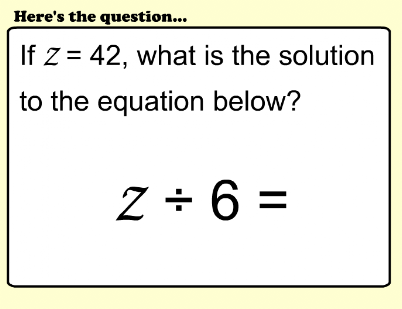5. Answer the question below. *
1 point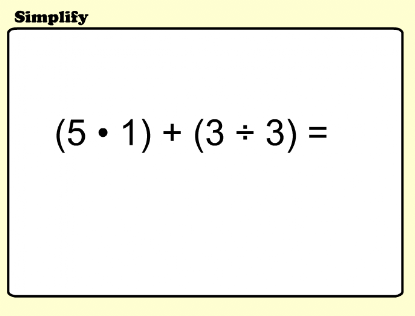6. Answer the question below. *
1 point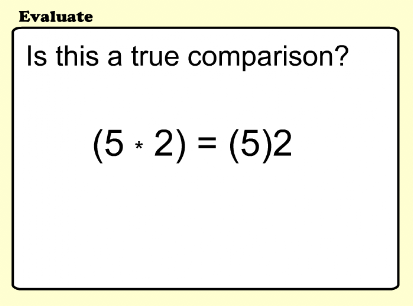7. Answer the question below. *
1 point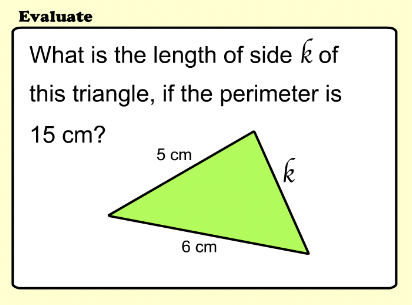8. Answer the question below. *
1 point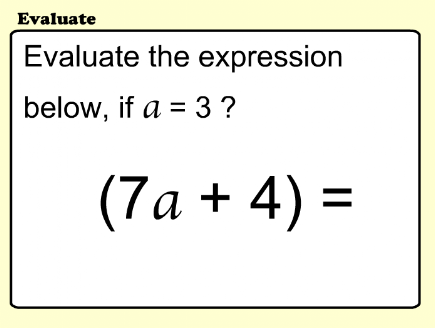9. Answer the question below. *
1 point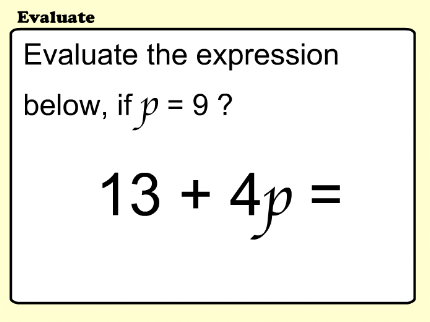10. Answer the question below. *
1 point